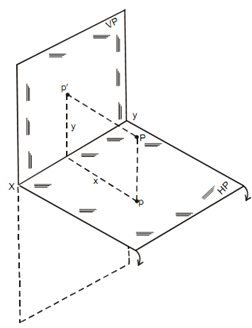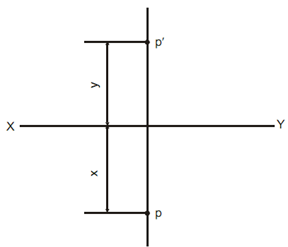## Projections of Point located in the First Quadrant Assignment Help

Assignment Help: >> Projection of Points - Projections of Point located in the First Quadrant

Projections of Point located in the First Quadrant:

The point P is located in first quadrant that is y units above HP and x units in front of VP while two projectors or imaginary lines one parallel to HP and other perpendicular to VP are drawn from this point P, they will intersect VP and HP at p′ and p respectively. These two points' p′ on VP and p on HP are the elevation and plan of point P.(a)(b)

The method of receiving the projection of P is as follows in diagram (b).

(a) Sketch a horizontal line of any type of length and mark it X-Y. This is called as reference line.

(a)   Sketch a thin and comparatively light line perpendicular to X-Y. This line is called as projector.

(b)   Mark a point p′, on the projection on a distance y from X-Y and above it.

(c)    Mark a point p on the projector on a distance x from X-Y and below it.

p′ and p are the projections of P in Ist quadrant.